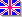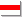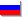### Statistics, diffusion processes and Brownian motion

#### Diffusion processes and Brownian motion

Let's consider the Brownian motion of some particles in ideal gas of particles of another sort supposing that concentration of the former is not very big, and we can regard their motion as a number of random transpositions. It means that while considering the motion of the particles of the first sort we can take into account only collisions with the second sort of particles and we can regard the distribution of the second sort of particles as uniform. Accepting such a suppositions we can obtain as the consequence that all points of space are equal for the particles of the first sort. It means that we can describe the motion of all the particles with fixed initial position in terms of conditional probabilities, taking all the possible pathways into account. (Statistical path integrals)

First of all, let's find out the average square of distance between the current position of some particle and it's initial position. (It's evident, that average radius-vector of the particle is equal to zero on principles of symmetry):
r2 = еi,jrirj = еiri2 + е i j rirj
But the latter sum is equal to zero because all transpositions are statistically independent. Finally:
<r2> = n*<D r2> = <D r2>*t /<D t>
Here <D r2> is the average free path and <D t> is the average time between the collisions of particles of different sorts. For simplicity we will write previous dependance in such a form:
<r2> = At
where A is some constant.

Finally, the integral conditions for required probability distribution of the particles in the space are:
p(r,t) = т...т p(D r0,D t0) p(D r1,D t1)... p(D rn,D tn) dr1dr2...drn-1
where
еi D ri = r - r0еi D ti = t - t0,
dr is a small volume element and n is the number of paths taken into account. This number must tend to infinity.

One of possible solutions of this integral equation is:
p(r) = B*Exp(C*(r - r0)2)
Here A and B can be obtained from normality condition and from the previously obtained equality for average square of distance:
p(r) = (1/pAt)Exp(-r2/At)
And exactly this solution satisfies our integral conditions! (It can be checked easily by considering only two integrals. Try to evaluate this:

Here is another approach to this problem of diffusion theory. Diffusion equation for small concentration is:
nv = D*grad n
where n is concentration, v is average velocity of ordered motion of particles and D is diffusion constant. Equation of continuity is:
div nv + dn/dt = 0
The result of two previous equations is:
DDn + dn/dt = 0
Here D is Laplacian. The functions
p(r) = (1/pAt)Exp(-r2/At)
are Green functions of this equation - it's solutions with delta-function as initial conditions. In our problem all the particles initially have the same positions, and their distribution are delta-function!

Real-time model of Brownian motion Brownian.zip

#### Percolation theory

Let's consider some crystal with structural defects, atoms of some other element. Let's accept, that electric current can't pass through the defect. When concentration of defects is greater than some critical value, the whole crystal becomes non-conductor. The main task of percolation theory is to determine this critical value for different configurations of crystal structures. When the concentration of defects is equal to this critical value, the slight change in concentration leads to the great change in conductivity. There is another interesting effect: when the concentration of defects is near to this critical value, the clusters of non-defect crystal cells are the fractal structures! For simple 2D grid the critical concentration of defects is 0.41, and for simple 3D grid - 0.69.

Here are the program source to view and explore 2D and 3D crystals, and to process the results of the experiments (defining the critical value, see Less Square Method)
Percol.zip

#### Gas outflow process in vacuum

Let's consider a container filled with ideal gas. There is a small hole in the container, so the gas is flowing out rather slowly. Let's find out how the temperature of the gas depends on time. All the parameters of any statistical system can be described with some distribution function. For example, if considered parameter is absolute value of velocity, then the number of particles with velocity from v to v + dv is:
dN = N*f(v)*dv
where N is the total number of particles in the system. It is evident that
тf(v)dv = 1
The function f(v) may change in time. Average velocity of particles in the system is:
<v> = тvf(v)dv
Average square of velocity is:
<v2> = тv2f(v)dv
This value can be considered as the temperature of the gas because kinetic energy of the particle in classic mechanics is proportional to the square of its velocity, and the temperature is proportional to average energy of particles in the system. Due to the hole in the container number of particles decreases, and the rate of change of number of particles with velocities from v to v + dv is:
d(dN)/dt = - dN*S*v/(4*V)
where S is the square of the hole and V is capacity of the container. Combining all the previous equations such an equation for f(v) can be obtained:
df(v)/dt = - A*f(v)*(v - <v>)
where A = V/(4*S). It is evident that particles with greater velocity are emitted from the container more intensively, and the maximum of f(v) is moved in the direction of decreasing velocities. Average velocity and average square of velocity (temperature) are also decreasing. It's quite an interesting statistical effect - the temperature of gas in the container decreases, but there are no direct interaction between particles and the container which can change the speed of the particles! It's also evident that the most frequently used for ideal gas Maxwell distribution can not be used when solving obtained equation. There is no surprize - Maxwell distribution is distribution for statical systems, and our system is not statical.

Here is the program, which models the gas outflow process in vacuum and evaluates the temperature of gas as the function of time  Gas.zip

#### Entropy

Entropy is the meausure of chaotic behaviour of any statistical assembly. An universal defintion for entropy is based on distribution function of statistical assembly, but not dS = DQ/T, which is most frequently used formala for entropy. Generally:
S = Si pi ln(1/pi)
where pi are the probabilities of statistically independent events 1..i. This defintion can be applied both to "heat" theory and information theory (coding theory). The most interesting experiment to prove this statement is:

Put your computer into thermostat. Fill the hard disk of your computer with random data and evaluate it's entropy, using distribution function for values of bytes. Then archive all the data. Measure the segregated heat Q and CPU temperature T. Evaluate entropy of your hard disk again. Is Q/T equal to change in HDD's entropy? ;))) I think it depends on frequency of your CPU :))))

Now let's prove such a well-known formulae for entropy:
(i) dS = DQ/T and
(ii) S = ln N
where DQ is infinitesimal amount of heat, T is thermodynamic temperature, N is the number of microstates within the given energy state. Formula (i) is special case of general formula applied to equilibrium Gibbs distribution. For this distribution
pi = A*Exp(-b*Ei)
where b = 1/(kT), Ei is energy in state i, A = 1/(Si Exp(-b*Ei))
After some mathematical work, such a formula can be derived from considered distribution and general formula:
dS = (<E2> - <E>2)b*db (*)
Note that all Ei are considered as constants for differentiation. But according to the law of conservation of energy:
d(<E>) = DQ(**)
Evaluating d(<E>), we obtain:
d(<E>) = (<E2> - <E>2)*db
Comparing this, (*) and (**), we prove the initial formula:
dS = DQ/T (constant multiplier k is not taken into account; it depends only on current system of units).
Formula (ii) is simply proved by ergodic hypothesis and Liouville theorem. According to them, all the microstates within the given energy state are equally probable, that is:
pi = 1/N,   i=1..N
Obviously
S = ln N (constant multiplier is not taken into account, too)

Finally, let's derive the formula for previously used Gibbs distribution. The value of entropy culminates in equilibrium state (And equilibrium is the most possible state for all the physical phenomena). All the probabilities must be varied to define position of extremum with additional conditions according to defintion of probability and the law of conservation of energy:
dS = Si d(pi ln(1/pi)) = Si dpi ln(1/pi) + Si pi dpi/pi = Si dpi ln(1/pi) = 0
Sipi = 1 or Sidpi = 0
Si Ei pi = <E> = const or Si Ei dpi = 0
Using Lagrange method to define position of extremum with additional conditions, we obtain:
Si (-ln pi + a*Ei + b)dpi = 0
where a, b are Lagrange multipliers. Therefore:
-ln pi = -a*Ei - b
pi = A1 Exp( - A2*Ei)
where A1, A2 are some constants. Our conditions declare that:
A2 = 1/T (constant multipliers are'nt taken into account as always:))

©2002-2003, VeterEnglishБеларускаяРусский
Сайт создан в системе uCoz